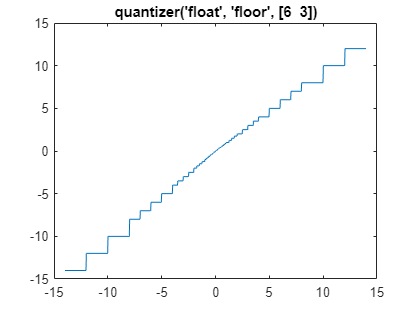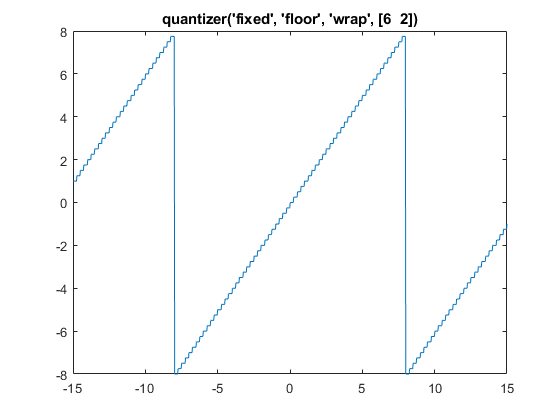# quantize method

Apply `quantizer` object to data

## Syntax

```y = quantize(q, x) [y1,y2,...] = quantize(q,x1,x2,...) ```

## Description

`y = quantize(q, x)` uses the `quantizer` object `q` to quantize `x`. When `x` is a numeric array, each element of `x` is quantized. When `x` is a cell array, each numeric element of the cell array is quantized. When `x` is a structure, each numeric field of `x` is quantized. Quantize does not change nonnumeric elements or fields of `x`, nor does it issue warnings for nonnumeric values. The output `y` is a built-in double. When the input `x` is a structure or cell array, the fields of `y` are built-in doubles.

`[y1,y2,...] = quantize(q,x1,x2,...)` is equivalent to `y1 = quantize(q,x1), y2 = quantize(q,x2),... `

The `quantizer` object states

• `max` — Maximum value before quantizing

• `min` — Minimum value before quantizing

• `noverflows` — Number of overflows

• `nunderflows` — Number of underflows

• `noperations` — Number of quantization operations

are updated during the call to `quantize`, and running totals are kept until a call to `resetlog` is made.

## Examples

collapse all

The following example demonstrates using `quantize` to quantize data.

```u=linspace(-15, 15, 1000); q=quantizer([6 3], 'float'); range(q)```
```ans = 1×2 -14 14 ```
`y=quantize(q, u);`
```Warning: 68 overflow(s) occurred in the fi quantize operation. ```
`plot(u, y); title(tostring(q))`The following example demonstrates using `quantize` to quantize data.

```u=linspace(-15, 15, 1000); q=quantizer([6 2], 'wrap'); range(q)```
```ans = 1×2 -8.0000 7.7500 ```
`y=quantize(q, u);`
```Warning: 468 overflow(s) occurred in the fi quantize operation. ```
`plot(u, y); title(tostring(q))`